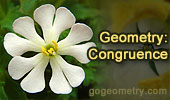# Online Geometry: Congruence, Rotation, Translation, Reflection - Page 8Proposed Problem 34.
Right triangle, cevian, incircles, tangents and inradius.

Problem 32.
Triangle, Cevian, Incircles, Tangents.

Problem 31. Right Triangle, Incircle, Collinears.
Problem 30. Right Triangle, Incircle, Inradius.

Proposed Problem 29.
Right Triangle, altitude, incircle and inradius. Ten conclusions.

Problem 29: Geometry Help.
Facts you should know.

Proposed Problem 28
Right Triangle, altitude, incircles and inradius.

Proposed Problem 26
Right Triangle, altitude, incircles and inradius.

Proposed Problem 25
Right Triangle, altitude, incircles and inradius.

Previous | 1 | 2 | 3 | 4 | 5 | 6 | 7 | 8 | 9 | 10 | Next

 Home | Geometry | Problems | Email | By Antonio Gutierrez